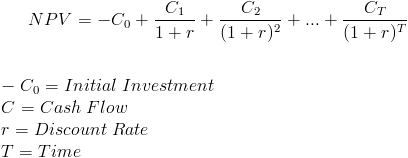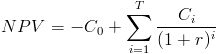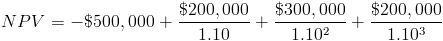# Net Present ValueNet Present Value(NPV) is a formula used to determine the present value of an investment by the discounted sum of all cash flows received from the project. The formula for the discounted sum of all cash flows can be rewritten asWhen a company or investor takes on a project or investment, it is important to calculate an estimate of how profitable the project or investment will be. In the formula, the -C0 is the initial investment, which is a negative cash flow showing that money is going out as opposed to coming in. Considering that the money going out is subtracted from the discounted sum of cash flows coming in, the net present value would need to be positive in order to be considered a valuable investment.

## Example of Net Present Value

To provide an example of Net Present Value, consider company Shoes For You's who is determining whether they should invest in a new project. Shoes for You's will expect to invest \$500,000 for the development of their new product. The company estimates that the first year cash flow will be \$200,000, the second year cash flow will be \$300,000, and the third year cash flow to be \$200,000. The expected return of 10% is used as the discount rate.

The following table provides each year's cash flow and the present value of each cash flow.

YearCash FlowPresent Value
0-\$500,000-\$500,000
1\$200,000\$181,818.18
2\$300,000\$247,933.88
3\$200,000\$150,262.96

Net Present Value = \$80,015.02

The net present value of this example can be shown in the formulaWhen solving for the NPV of the formula, this new project would be estimated to be a valuable venture.

New to Finance?